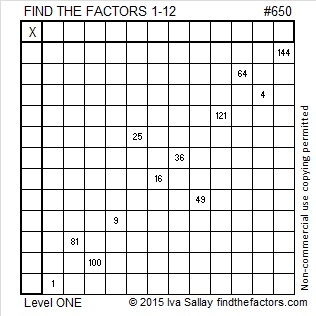# 650 is the sum of all the clues in this Level 1 puzzle

1² + 2² + 3² + 4² + 5² + 6² +7² + 8² + 9² +10² + 11² + 12²  = 650

Thus 650 is the 12th square pyramidal number and can be calculated using 12(12 +1)(2⋅12 + 1)/6.

If you add up all the clues in today’s Find the Factors puzzle, you will get the number 650. However, if you print the puzzle from the excel file, one of the clues is missing because it isn’t needed to find the solution.

650 is the hypotenuse of seven Pythagorean triples!

• 72-646-650
• 160-630-650
• 182-624-650
• 250-600-650
• 330-560-650
• 390-520-650
• 408-506-650

Can you find the greatest common factor of each triple? Each greatest common factor will be one of the factors of 650 listed below the puzzle.

650 is the hypotenuse of so many Pythagorean triples because it is divisible by 5, 13, 25, 65, and 325 which are also hypotenuses of triples. The smallest three numbers to be the hypotenuses of at least 7 triples are 325, 425, and 650.

Since 25 x 26 = 650, we know that (25-1)(26 + 1) = 650 – 2. Thus 24 x 27 = 648.Print the puzzles or type the solution on this excel file: 12 Factors 2015-10-19

—————————————————————————————————

• 650 is a composite number.
• Prime factorization: 650 = 2 x 5 x 5 x 13, which can be written 650 = 2 x (5^2) x 13
• The exponents in the prime factorization are 1, 2, and 1. Adding one to each and multiplying we get (1 + 1)(2 + 1)(1 + 1) = 2 x 3 x 2 = 12. Therefore 650 has exactly 12 factors.
• Factors of 650: 1, 2, 5, 10, 13, 25, 26, 50, 65, 130, 325, 650
• Factor pairs: 650 = 1 x 650, 2 x 325, 5 x 130, 10 x 65, 13 x 50, or 25 x 26
• Taking the factor pair with the largest square number factor, we get √650 = (√25)(√26) = 5√26 ≈ 25.495098.—————————————————————————————————

## 2 thoughts on “650 is the sum of all the clues in this Level 1 puzzle”

1.Joseph Nebus

Nice pyramidal number here. Thanks.

•ivasallay

It’s always a pleasure.

This site uses Akismet to reduce spam. Learn how your comment data is processed.